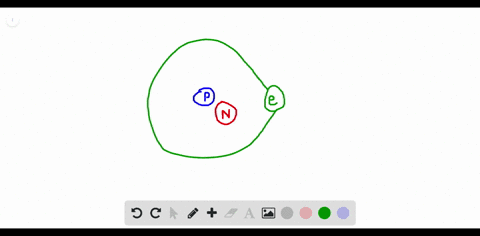### How can you determine the number of neutrons in a…

00:20University of Miami
Problem 60

How is the mass number related to the number of protons and neutrons an atom has?

The mass number is the number of protons and neutrons.

## Discussion

You must be signed in to discuss.

## Video Transcript

So now we'll work on problems 60 from Chapter four. This problem were asked about the mass number and how it relates to the number of protons and neutrons set and Adam hats. So these two are actually closely related because the mass number is equal to the sum of neutrons and protons in an Adam, so that is the definition of mass numbers, so